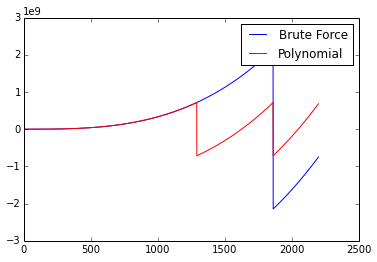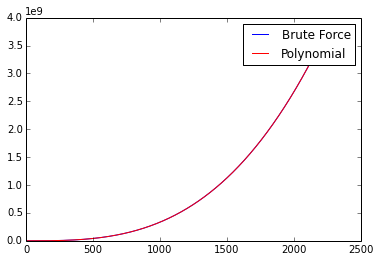# Project Euler Problems 5-6

## Problem 5¶

2520 is the smallest number that can be divided by each of the numbers from 1 to 10 without any remainder.

What is the smallest positive number that is evenly divisible by all of the numbers from 1 to 20?

This is an interesting problem!

First thing's first, we can establish that the largest positive number that meets the condition is $1×2×3..×20$ or simply $20!$ We can work our way down by repeatedly dividing this upper boundary number by any number in the range [1,20] and seeing if it's an even division.

This approach results in a runtime complexity of O(log(n!)), better known as O(n log n)

In :
factors = 20

upper = math.factorial(factors)
divisors = range(2, factors+1)
current = upper

#repeatedly attempt to divide current number by prime factors ordered
#from largest to smallest as long as the result has a remainder of 0
while True:
found = False
for p in reversed(divisors):
c = current / p
if c % p == 0:
found = True
current = c
break

break

print 'divided by', p, 'got', current

divided by 20 got 121645100408832000
divided by 20 got 6082255020441600
divided by 20 got 304112751022080
divided by 18 got 16895152834560
divided by 18 got 938619601920
divided by 18 got 52145533440
divided by 16 got 3259095840
divided by 14 got 232792560
divided by 12 got 19399380
divided by 2 got 9699690



## Problem 6¶

The sum of the squares of the first ten natural numbers is, 12 + 22 + ... + 102 = 385

The square of the sum of the first ten natural numbers is, (1 + 2 + ... + 10)2 = 552 = 3025

Hence the difference between the sum of the squares of the first ten natural numbers and the square of the sum is 3025 − 385 = 2640.

Find the difference between the sum of the squares of the first one hundred natural numbers and the square of the sum.

Method 1: brute force

Complexity: O(N)

In :
def squareDiff(x):
s = range(1, x+1)
sumSquares = sum([x*x for x in s])
squareSum = math.pow(sum(s),2)
diff = squareSum - sumSquares
return diff

squareDiff(100)

Out:
25164150.0


Easy enough, however it's well known that the sum of a series of natural numbers up to n can be calculated as $\frac{n(n+1)}{2}$

Is it possible that the sum of a series of natural numbers squared up to n can be calculated in constant time as well? I didn't know the answer and cheated by using a genetic algorithm to attempt to fit an equation to match sumSquares for the first 140 inputs.

Amazingly, it came back with a polynomial that had 0 residual error: $\frac{1}{6}n + \frac{1}{2}n^2 + \frac{1}{3}n^3$

Let's plot this polynomial to double check

In :
brute = lambda n: sum([x*x for x in xrange(1,n+1)])
poly = lambda n: round(1./6 * n + 1./2 * pow(n, 2) + 1./3 * pow(n, 3))

x = np.array(range(1,2200))
brute_y = np.array([brute(t) for t in x])
poly_y = np.array([poly(t) for t in x])

plt.plot(x, brute_y, label='Brute Force', color='blue')
plt.plot(x, poly_y, label='Polynomial', color='red')
plt.legend()

print 'max error:', max(brute_y - poly_y)

max error: 1431655765.0Looks like the polynomial solution suffers from integer overflow at around n$\approx$1300; earlier than the brute force solution. This is understandable considering the polynomial solution deals with n3 while brute force only deals with n2. We'll switch to floats to overcome overflow issues in both cases.

In :
brute = lambda n: sum([1.*x*x for x in xrange(1,n+1)])
poly = lambda n: round(1./6 * n + 1./2 * pow(n, 2.) + 1./3 * pow(n, 3.))

x = np.array(range(1,2200))
brute_y = np.array([brute(t) for t in x])
poly_y = np.array([poly(t) for t in x])

plt.plot(x, brute_y, label='Brute Force', color='blue')
plt.plot(x, poly_y, label='Polynomial', color='red')
plt.legend()

print 'max error:', max(brute_y - poly_y)

max error: 0.0A maximum error of 0 across a small input set is promising, however I got in touch with a friend to check, and he promtly came back with a proof!

All credit to Jonah Schreiber for the below proof:

$\sum_{x=1}^{n}{x^2}=\frac{n^3}{3}+\frac{n^2}{2}+\frac{n}{6}$

Base

Here, we show that the formula is correct for $n=1$. On the left-hand side, we have $1$, and on the right-hand side, we have $\frac{1^3}{3}+\frac{1^2}{2}+\frac{1}{6}=1$, so the base case is true.

Assumption

Assume that

$\sum_{x=1}^{n}{x^2}=\frac{n^3}{3}+\frac{n^2}{2}+\frac{n}{6}$

is true.

Induction

Show then that it is true for $n+1$, that is,

$\sum_{x=1}^{n+1}{x^2}=\frac{(n+1)^3}{3}+\frac{(n+1)^2}{2}+\frac{n+1}{6}$

Let us break out the last term on the left-hand side, and expand the right-hand side:

$\sum_{x=1}^{n}{x^2}+(n+1)^2=\frac{n^3+3n^2+3n+1}{3}+\frac{n^2+2n+1}{2}+\frac{n+1}{6}$

We already know the sum on the left-hand side, which we insert.

$\frac{n^3}{3}+\frac{n^2}{2}+\frac{n}{6}+n^2+2n+1=\frac{n^3+3n^2+3n+1}{3}+\frac{n^2+2n+1}{2}+\frac{n+1}{6}$

Collecting terms, we get

$\frac{n^3}{3}+\frac{3n^2}{2}+\frac{13n}{6}+1=\frac{n^3}{3}+\frac{3n^2}{2}+\frac{13n}{6}+1$

The two sides are equal, so the formula is correct.

Thanks again to Jonah Schreiber for the above proof.

See http://www.trans4mind.com/personal_development/mathematics/series/sumNaturalSquares.htm for several derivations of this formula.

And so, finally,

Method 2: Arithmetic

Complexity: O(1)

In :
def squareDiff2(x):
sumSquares = round(1./6 * x + 1./2 * pow(x, 2.) + 1./3 * pow(x, 3.))
squareSum = pow(x*(x+1)/2., 2)
return squareSum - sumSquares

squareDiff2(100)

Out:
25164150.0